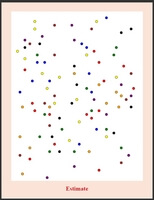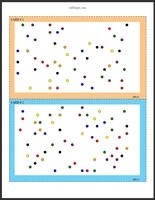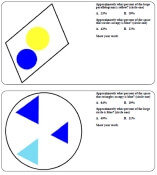Worksheets and No Prep Teaching Resources
Math Worksheets

# Rounding Worksheets

Estimating things, from how long a task will take to how many apples are needed for a pie, is a regular part of practical life. When students practice this skill, it gives them a down-to-earth advantage in the future. Whether its length, weight, time, or quantities, students grade one through six will get lots of fascinating practice in estimating with these innovative worksheets. Rounding numbers is a related skill, and students will become experts in rounding both whole numbers and decimals with these thorough worksheets that can be customized to individual students' skill levels.

Length and Weight (level: grade 1)
Length (black and white graphics)
Estimate (inches and feet)
Estimate (centimeters and meters)
Weight (black and white graphics)
Estimate (ounces and pounds)
Estimate (grams and kilograms)

Length (color graphics)
Estimate (inches and feet)
Estimate (centimeters and meters)
Weight (color graphics)
Estimate (ounces and pounds)
Estimate (grams and kilograms)

How long will it take? (estimating the time needed to do something; level: grade 2)
Comparing things to do to one minute
Comparing things to do to 30 minutes
Mixed

Color Gumballs Math Learning Center: Estimate - Match Picture of Gumballs with EstimateGumballs: Estimate by tens - 10, 20, up to 100 - Ten Cards - Match Estimate with Picture (Grades 2-6) Gumballs: Estimate by tens - Five Cards (Grades 2-6)

Black and White Rectangles to Estimate - Math Learning Center: Match Picture with EstimateEstimate by tens - 10, 20, up to 100 - Ten Cards - Match Estimate with Picture (Grades 2-6) Estimate by tens - Five Cards (Grades 2-6)

Writing an Estimate - Math Learning CenterTens: Writing an Estimate - Ten Cards (Grades 4-6) Tens: Writing an Estimate - Five Cards (Grades 4-6)

Rounding Whole Numbers (level: grades 3-6)
Round to the nearest ten
Round to the nearest hundred
Round to the nearest thousand
Write the 2 tens between each number
Write the 2 hundreds between each number
Write the 2 thousands between each number
Round to the place of the underlined digit (tens, hundreds, and thousands)
Fill in the missing digit (ones, tens, or hundreds digits blank)
Fill in the missing digit (tens, hundreds, or thousands digits blank)
Fill in the missing digit (hundreds, thousands, or ten-thousands digits blank)
Round to the nearest ten-thousand
Round to the nearest hundred-thousand
Round to the nearest million
Round to the place of the underlined digit (ten-thousands, hundred-thousands, and millions)
Round to the place of the underlined digit (ones through millions)
Rounding Whole Numbers Mixed Review

Rounding Money (level: grades 3-6)
Round to the nearest dollar
Round to the nearest ten dollars
Round to the place of the underlined digit

Rounding Decimals (level: grades 4-6)
Rounding: Round to the nearest tenth
Rounding: Round to the nearest hundredth
Rounding: Round to the place of the underlined digit

Perfect Squares and Square Roots (level: grade 6)
Estimate a square root (multiple choice)

Percents and Estimation:  Estimate and Percents Shape PuzzleEstimate and Percents Shape Puzzle (requires knowledge of percents - grades 6+)

Have a suggestion or would like to leave feedback?
Leave your suggestions or comments about edHelper!